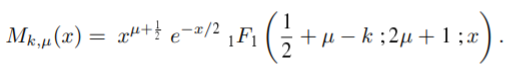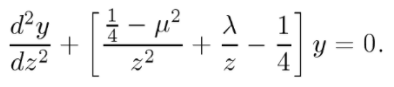# Whittaker Function

When Whittaker (1903) introduced his Whittaker function, he wrote it in terms of the closely related confluent hypergeometric function (Pearson et al., 2014; Ghayasuddin & Khan, 2017):The function is therefore sometimes called the confluent hypergeometric function, but it could more correctly described as a modification of those functions. Whittaker’s purpose in formulating the new function was to make formulas more symmetric.

If you’re interested, Whittaker’s original 1903 text is freely available via Project Euclid (downloadable PDF).

## Use of Whittaker Functions

The Whittaker function is a solution to the differential equation(White, 2010).
In mathematical physics, many functions are special cases of Whittaker functions, including error functions and the incomplete gamma function (White, 2010). Specific types include:

• Jacquet’s Whittaker functions: Generalized Whittaker functions of reductive groups over local fields,
• Kostant’s Whittaker functions: common eigenfunctions of Toda lattice Hamiltonians (Kostant, 1978).

In representation theory (which is, roughly speaking, the study of symmetry in linear spaces), the Whittaker function is well-studied example of a matrix coefficient of complex representations of p-adic algebraic groups. These appear in places like the integral expressions used to construct automorphic L-functions (Grodzicki, 2020). If you’re comfortable with representation theory of GLn(C), Dhillon’s The Models of Whittaker and Kirillov (PDF) is a good read on why you would want to consider Whittaker models.

## References

Dhillon, G. (2017). The Models of Whittaker and Kirillov. Retrieved September 26, 2020 from: http://virtualmath1.stanford.edu/~conrad/conversesem/Notes/L6.pdf
Ghayasuddin, M. & Khan, N. (2017). Some Transformations and Generating Relations of Multivariables Hypergeometric Functions. Palestine Journal of Mathematics. Vol 6 (Special Issue II).
Grodzicki, W. (2020). Wellesley College Bio: https://www.wellesley.edu/math/faculty/grodzickiw
Kostant, B. (1978). On Whittaker vectors and representation theory. Inventiones mathematicae volume 48, pages 101–184. Springer.
Pearson, J. et al., (2014). Numerical Methods for the Computation of the Confluent and Gauss Hypergeometric Functions.
White, R. (2010). Asymptotic Analysis of Differential Equations. Imperial College Press.
Whittaker, E. (1903). An expression of certain known function as generalized hypergeometric functions, Bull. Amer. Math. Soc, 10 (1903), 125-134

CITE THIS AS:
Stephanie Glen. "Whittaker Function" From StatisticsHowTo.com: Elementary Statistics for the rest of us! https://www.statisticshowto.com/whittaker-function/